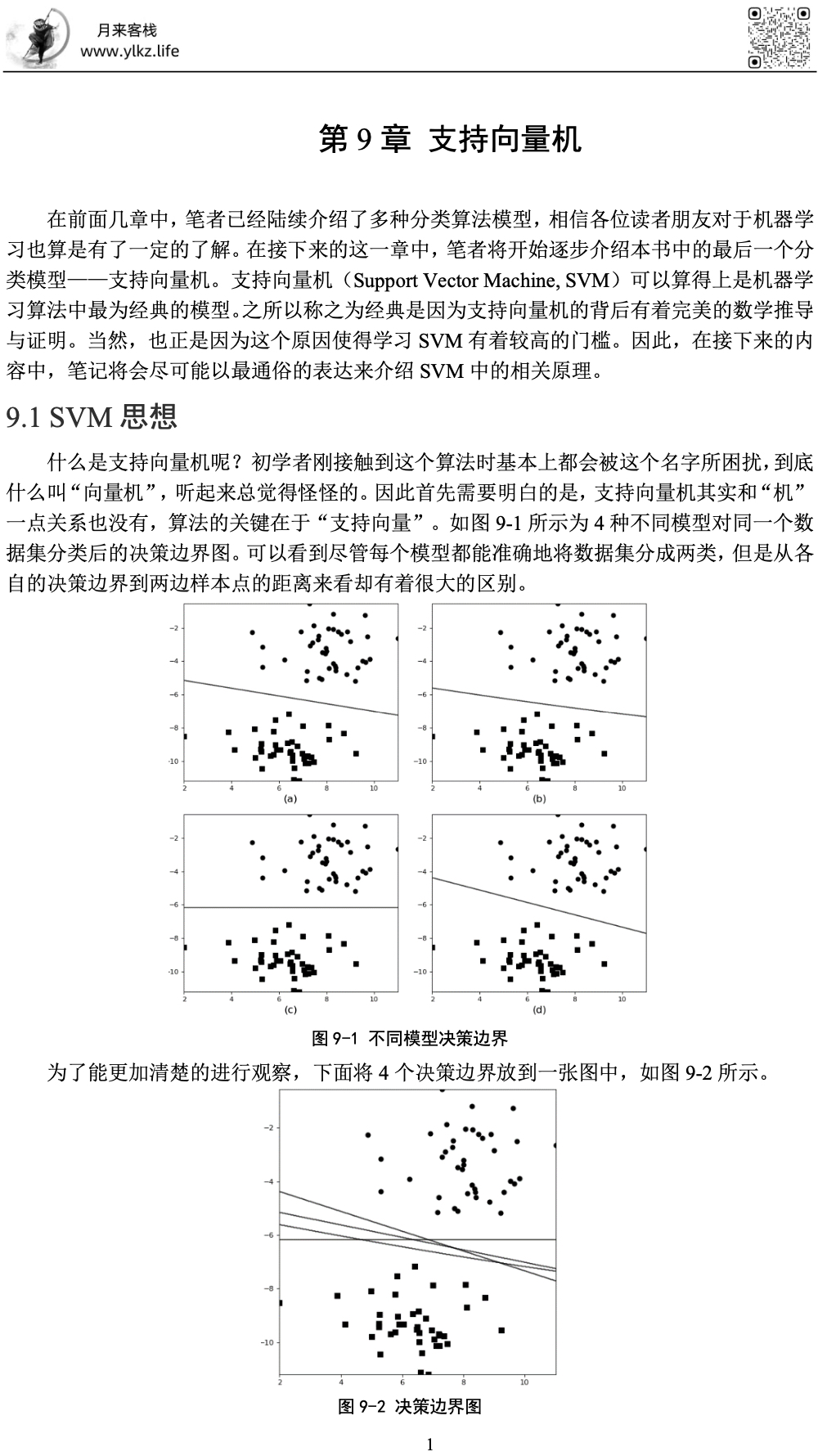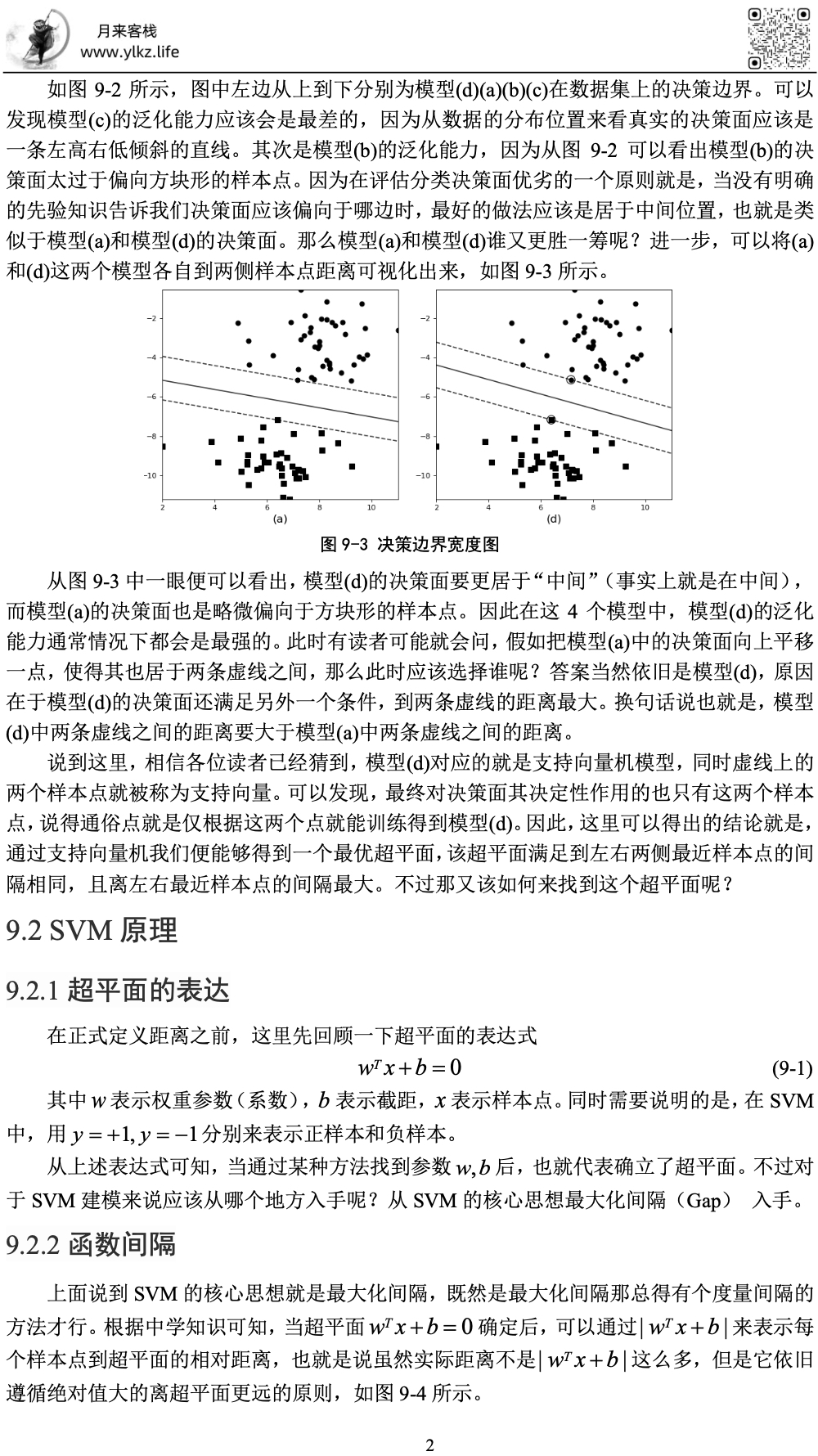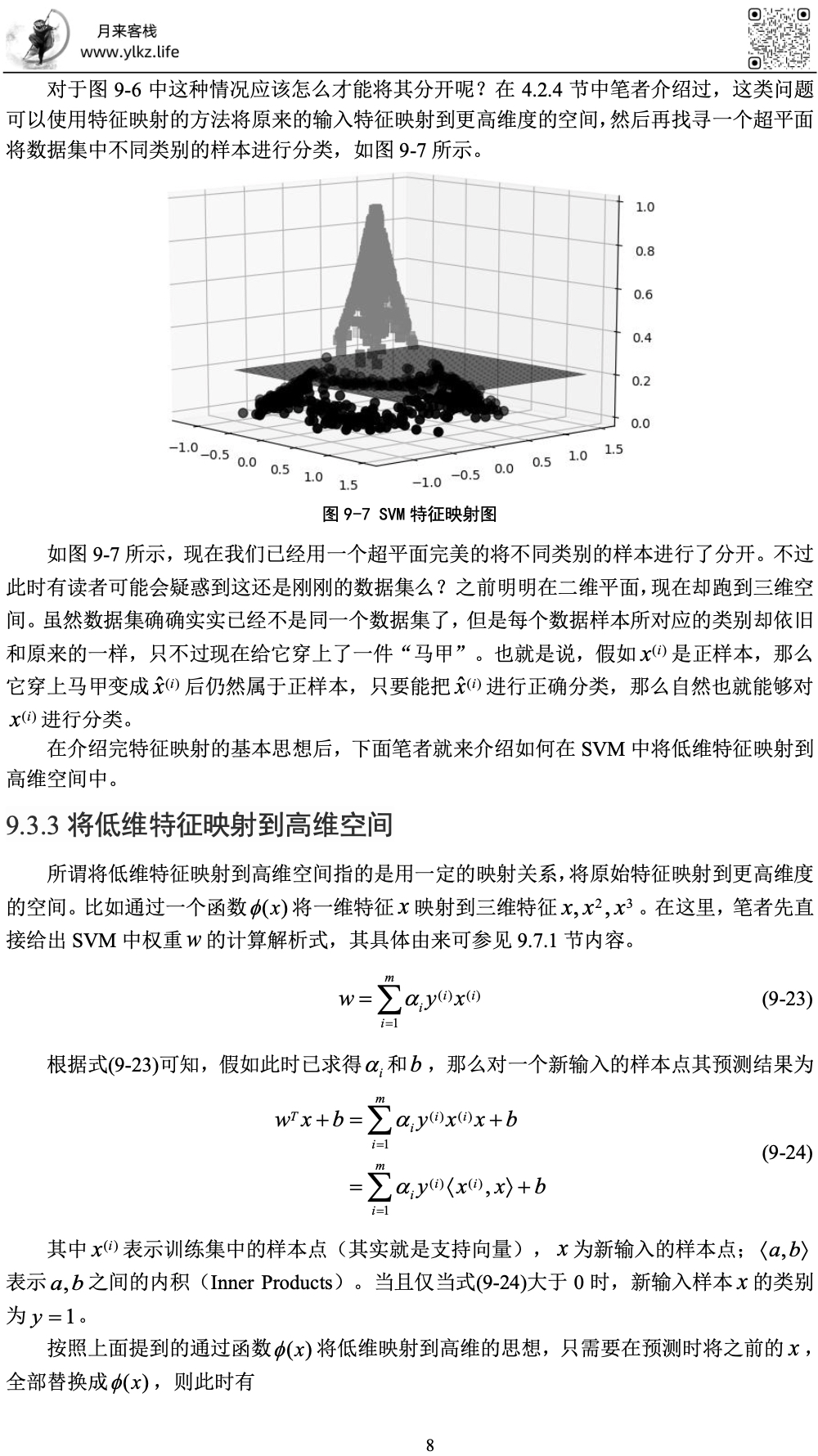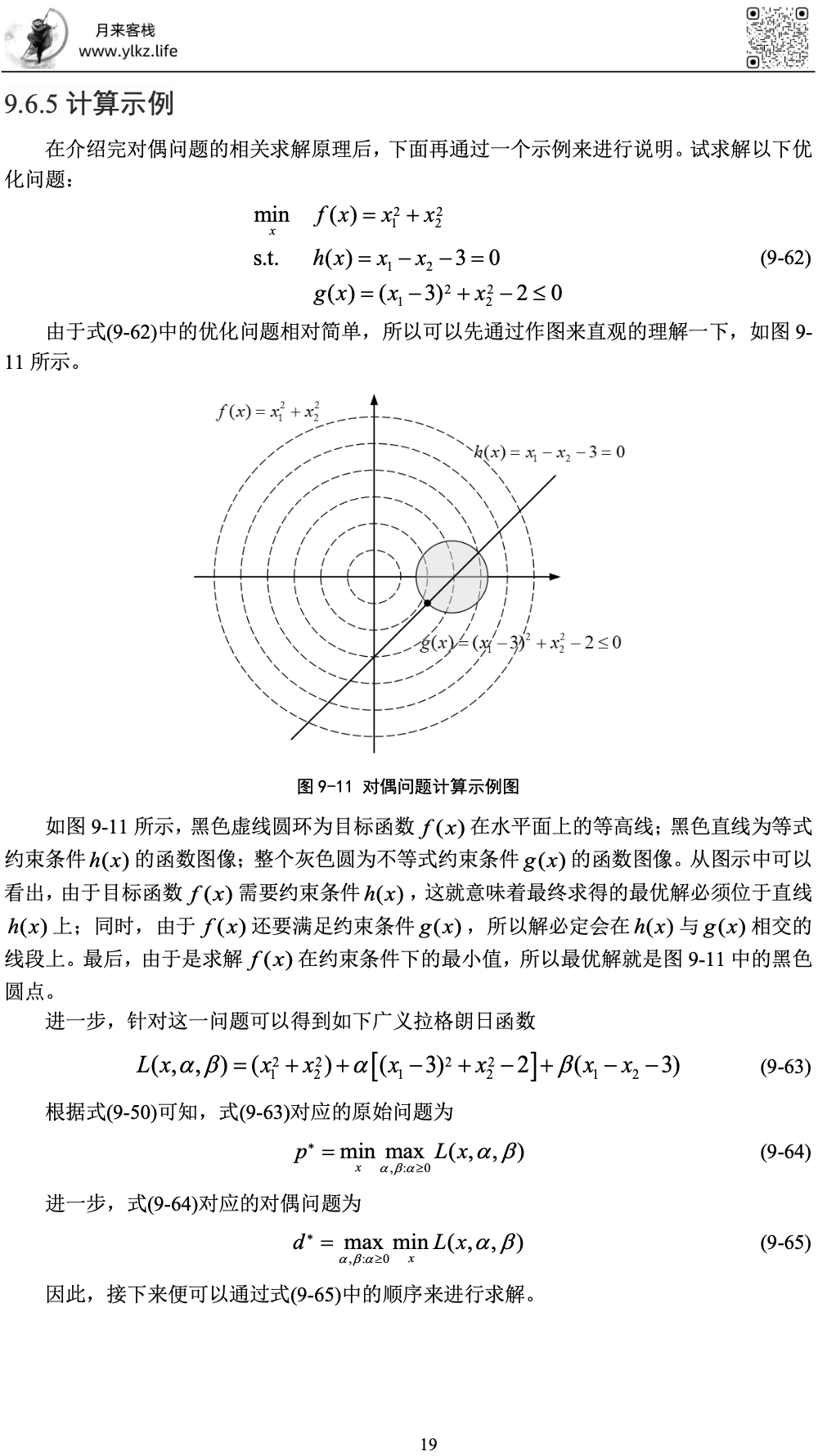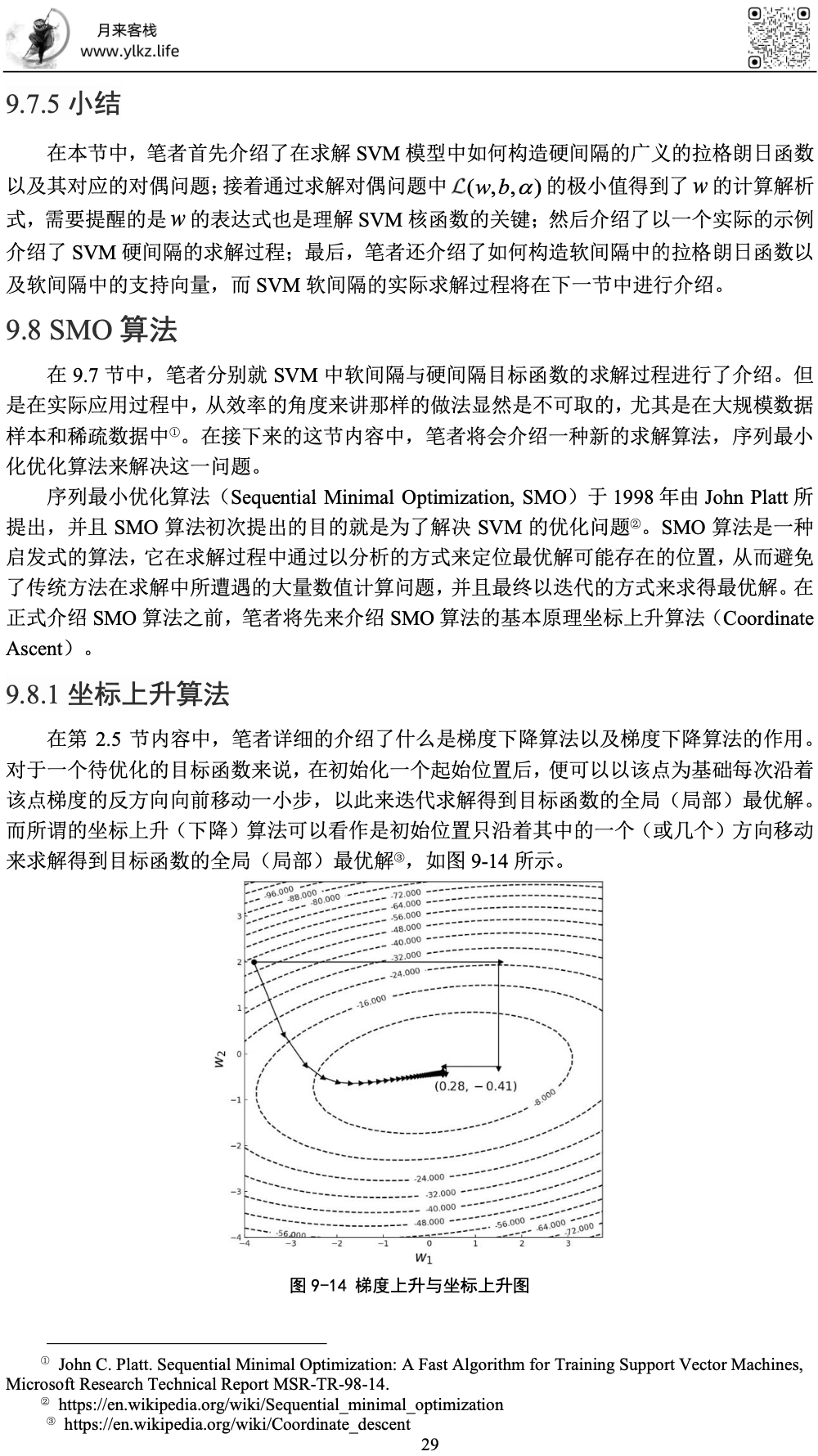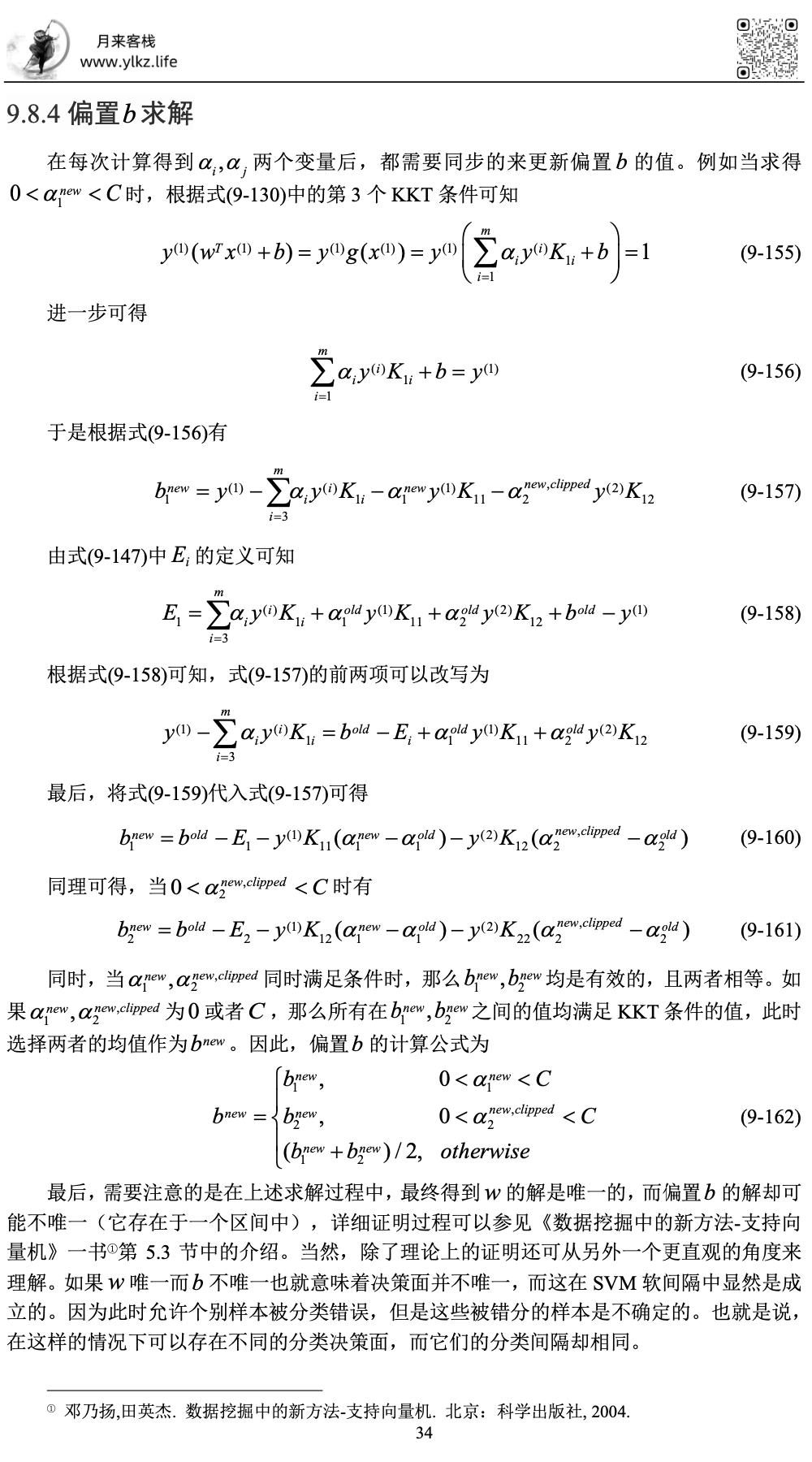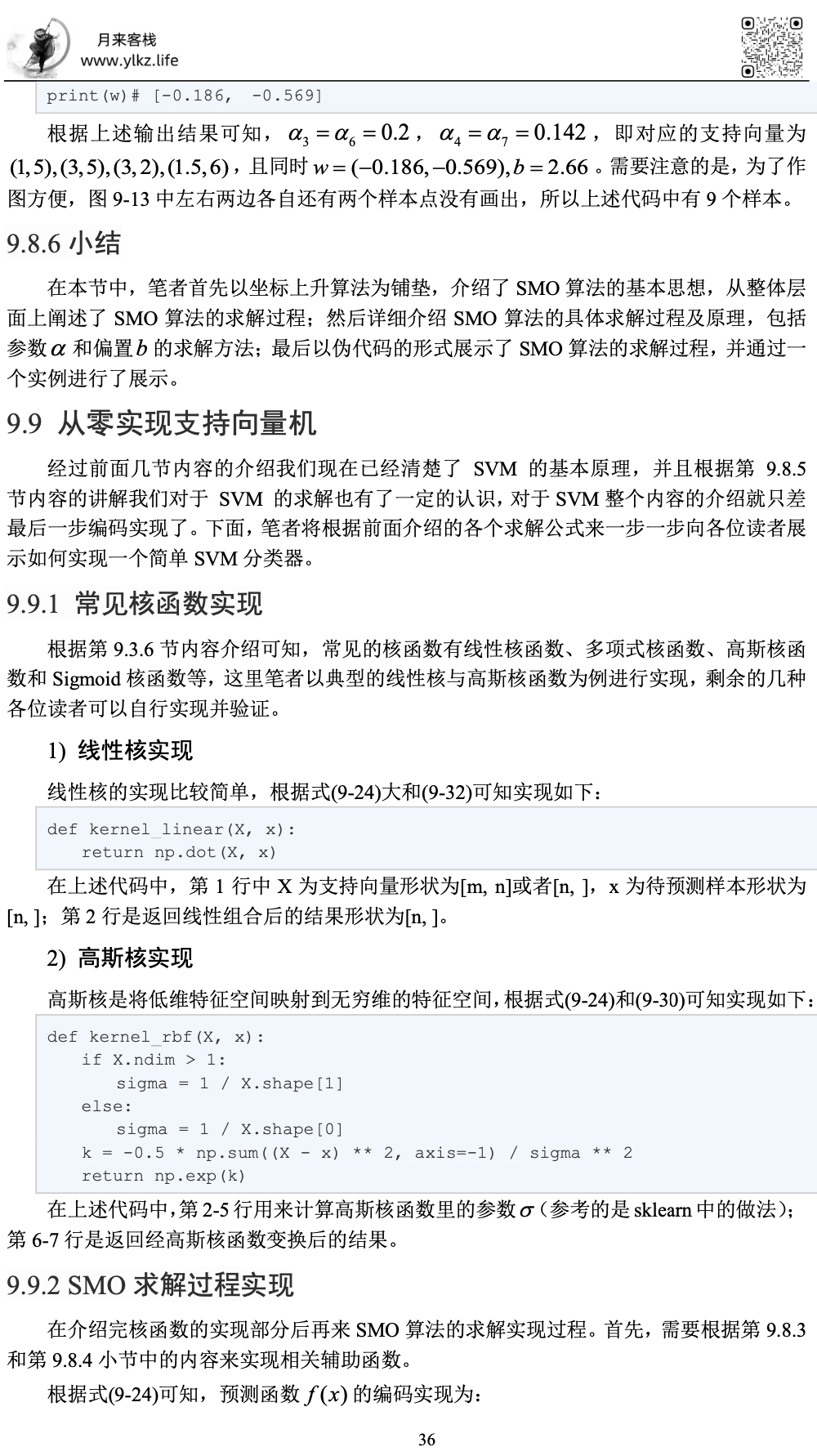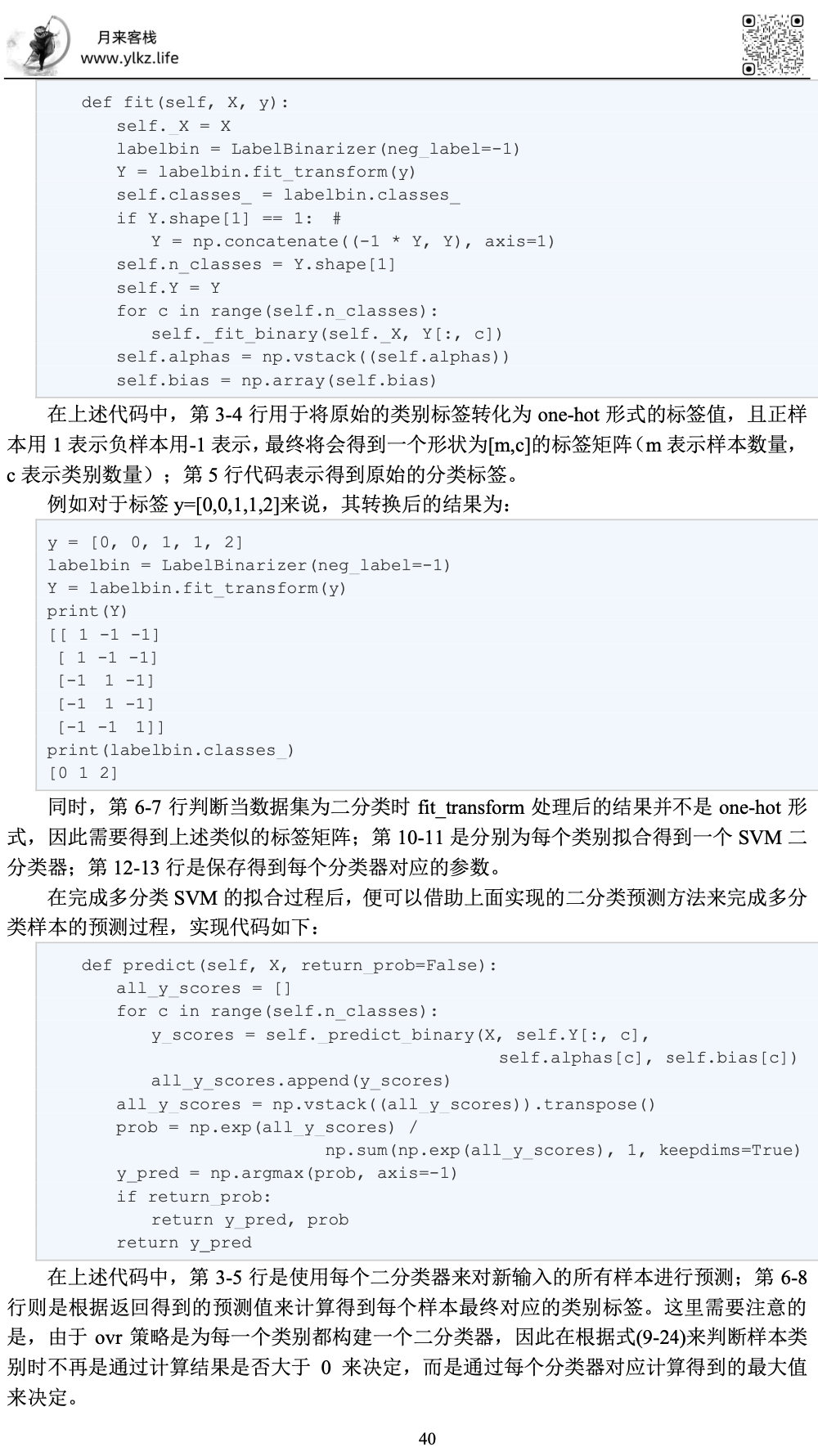### 9.1 SVM思想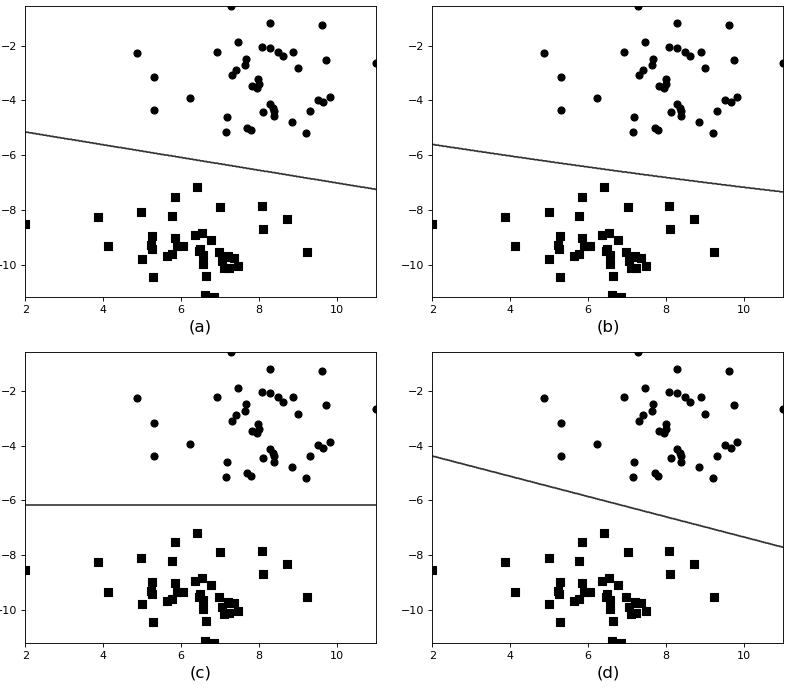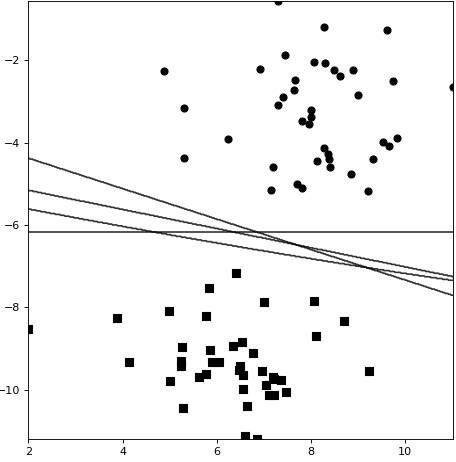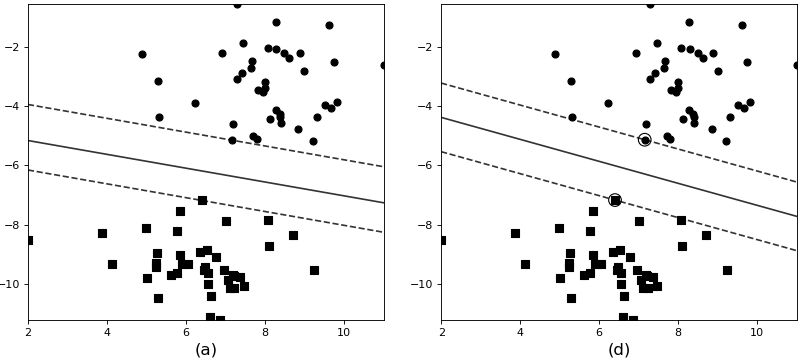### 9.2 SVM原理

#### 9.2.1超平面的表达

${w}^{T}x+b=0\phantom{\rule{0.278em}{0ex}}\phantom{\rule{0.278em}{0ex}}\phantom{\rule{0.278em}{0ex}}\phantom{\rule{0.278em}{0ex}}\phantom{\rule{0.278em}{0ex}}\phantom{\rule{0.278em}{0ex}}\phantom{\rule{0.278em}{0ex}}\phantom{\rule{0.278em}{0ex}}\phantom{\rule{0.278em}{0ex}}\phantom{\rule{0.278em}{0ex}}\left(9.1\right)$

#### 9.2.2函数间隔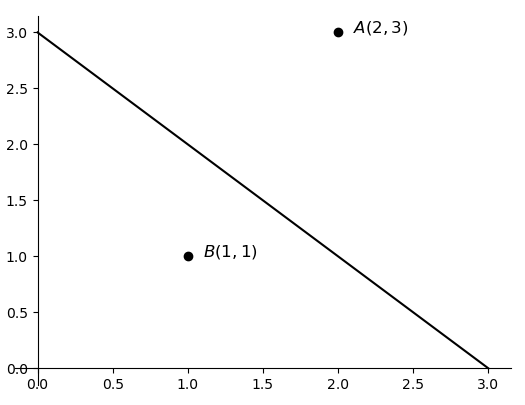${\stackrel{^}{\gamma }}^{\left(i\right)}={y}^{\left(i\right)}\left({w}^{T}{x}^{\left(i\right)}+b\right)\phantom{\rule{0.278em}{0ex}}\phantom{\rule{0.278em}{0ex}}\phantom{\rule{0.278em}{0ex}}\phantom{\rule{0.278em}{0ex}}\phantom{\rule{0.278em}{0ex}}\phantom{\rule{0.278em}{0ex}}\phantom{\rule{0.278em}{0ex}}\phantom{\rule{0.278em}{0ex}}\phantom{\rule{0.278em}{0ex}}\phantom{\rule{0.278em}{0ex}}\left(9.2\right)$

$\stackrel{^}{\gamma }=\underset{i=1,2,...,m}{min}\phantom{\rule{0.167em}{0ex}}{\stackrel{^}{\gamma }}^{\left(i\right)}\phantom{\rule{0.278em}{0ex}}\phantom{\rule{0.278em}{0ex}}\phantom{\rule{0.278em}{0ex}}\phantom{\rule{0.278em}{0ex}}\phantom{\rule{0.278em}{0ex}}\phantom{\rule{0.278em}{0ex}}\phantom{\rule{0.278em}{0ex}}\phantom{\rule{0.278em}{0ex}}\phantom{\rule{0.278em}{0ex}}\phantom{\rule{0.278em}{0ex}}\left(9.3\right)$

#### 9.2.3 几何间隔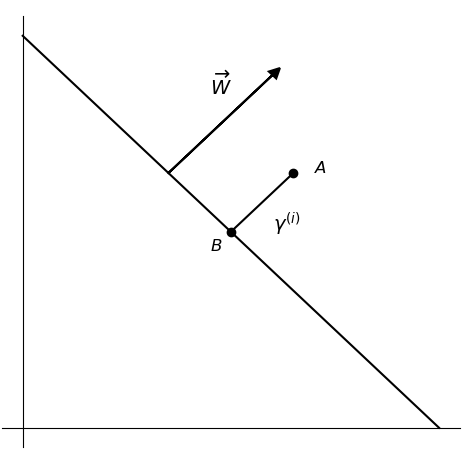${x}^{\left(i\right)}-{\gamma }^{\left(i\right)}\cdot \frac{W}{||W||}\phantom{\rule{0.278em}{0ex}}\phantom{\rule{0.278em}{0ex}}\phantom{\rule{0.278em}{0ex}}\phantom{\rule{0.278em}{0ex}}\phantom{\rule{0.278em}{0ex}}\phantom{\rule{0.278em}{0ex}}\phantom{\rule{0.278em}{0ex}}\phantom{\rule{0.278em}{0ex}}\phantom{\rule{0.278em}{0ex}}\phantom{\rule{0.278em}{0ex}}\left(9.4\right)$

${w}^{T}\left({x}^{\left(i\right)}-{\gamma }^{\left(i\right)}\cdot \frac{W}{||W||}\right)+b=0\phantom{\rule{0.278em}{0ex}}\phantom{\rule{0.278em}{0ex}}\phantom{\rule{0.278em}{0ex}}\phantom{\rule{0.278em}{0ex}}\phantom{\rule{0.278em}{0ex}}\phantom{\rule{0.278em}{0ex}}\phantom{\rule{0.278em}{0ex}}\phantom{\rule{0.278em}{0ex}}\phantom{\rule{0.278em}{0ex}}\phantom{\rule{0.278em}{0ex}}\left(9.5\right)$

${w}^{T}\left({x}^{\left(i\right)}-{\gamma }^{\left(i\right)}\cdot \frac{w}{||w||}\right)+b=0\phantom{\rule{0.278em}{0ex}}\phantom{\rule{0.278em}{0ex}}\phantom{\rule{0.278em}{0ex}}\phantom{\rule{0.278em}{0ex}}\phantom{\rule{0.278em}{0ex}}\phantom{\rule{0.278em}{0ex}}\phantom{\rule{0.278em}{0ex}}\phantom{\rule{0.278em}{0ex}}\phantom{\rule{0.278em}{0ex}}\phantom{\rule{0.278em}{0ex}}\left(9.6\right)$

${\gamma }^{\left(i\right)}=\frac{{w}^{T}{x}^{\left(i\right)}+b}{||w||}={\left(\frac{w}{||w||}\right)}^{T}{x}^{\left(i\right)}+\frac{b}{||w||}\phantom{\rule{0.278em}{0ex}}\phantom{\rule{0.278em}{0ex}}\phantom{\rule{0.278em}{0ex}}\phantom{\rule{0.278em}{0ex}}\phantom{\rule{0.278em}{0ex}}\phantom{\rule{0.278em}{0ex}}\phantom{\rule{0.278em}{0ex}}\phantom{\rule{0.278em}{0ex}}\phantom{\rule{0.278em}{0ex}}\phantom{\rule{0.278em}{0ex}}\left(9.7\right)$

${\gamma }^{\left(i\right)}={y}^{\left(i\right)}\left({\left(\frac{w}{||w||}\right)}^{T}{x}^{\left(i\right)}+\frac{b}{||w||}\right)\phantom{\rule{0.278em}{0ex}}\phantom{\rule{0.278em}{0ex}}\phantom{\rule{0.278em}{0ex}}\phantom{\rule{0.278em}{0ex}}\phantom{\rule{0.278em}{0ex}}\phantom{\rule{0.278em}{0ex}}\phantom{\rule{0.278em}{0ex}}\phantom{\rule{0.278em}{0ex}}\phantom{\rule{0.278em}{0ex}}\phantom{\rule{0.278em}{0ex}}\left(9.8\right)$

$\begin{array}{rl}& {\gamma }^{A}=+1\cdot \left({\left(\frac{w}{||w||}\right)}^{T}{x}^{\left(A\right)}+\frac{b}{||w||}\right)={\left(\frac{\left(1,1\right)}{\sqrt{1+1}}\right)}^{T}\left(2,3\right)+\frac{-3}{\sqrt{1+1}}=\sqrt{2}\\ & {\gamma }^{B}=-1\cdot \left({\left(\frac{w}{||w||}\right)}^{T}{x}^{\left(A\right)}+\frac{b}{||w||}\right)=-{\left(\frac{\left(1,1\right)}{\sqrt{1+1}}\right)}^{T}\left(1,1\right)+\frac{3}{\sqrt{1+1}}=\frac{1}{\sqrt{2}}\end{array}\phantom{\rule{0.278em}{0ex}}\phantom{\rule{0.278em}{0ex}}\phantom{\rule{0.278em}{0ex}}\phantom{\rule{0.278em}{0ex}}\phantom{\rule{0.278em}{0ex}}\phantom{\rule{0.278em}{0ex}}\phantom{\rule{0.278em}{0ex}}\phantom{\rule{0.278em}{0ex}}\phantom{\rule{0.278em}{0ex}}\phantom{\rule{0.278em}{0ex}}\left(9.9\right)$

$\gamma =\underset{i=1,2,...,m}{min}\phantom{\rule{0.167em}{0ex}}{\gamma }^{\left(i\right)}\phantom{\rule{0.278em}{0ex}}\phantom{\rule{0.278em}{0ex}}\phantom{\rule{0.278em}{0ex}}\phantom{\rule{0.278em}{0ex}}\phantom{\rule{0.278em}{0ex}}\phantom{\rule{0.278em}{0ex}}\phantom{\rule{0.278em}{0ex}}\phantom{\rule{0.278em}{0ex}}\phantom{\rule{0.278em}{0ex}}\phantom{\rule{0.278em}{0ex}}\left(9.10\right)$

$\gamma =\frac{\stackrel{^}{\gamma }}{||w||}\phantom{\rule{0.278em}{0ex}}\phantom{\rule{0.278em}{0ex}}\phantom{\rule{0.278em}{0ex}}\phantom{\rule{0.278em}{0ex}}\phantom{\rule{0.278em}{0ex}}\phantom{\rule{0.278em}{0ex}}\phantom{\rule{0.278em}{0ex}}\phantom{\rule{0.278em}{0ex}}\phantom{\rule{0.278em}{0ex}}\phantom{\rule{0.278em}{0ex}}\left(9.11\right)$

#### 9.2.4 最大间隔分类器

$\gamma$$\gamma$尽可能大，因为目的就是最大化$\gamma$$\gamma$;

② 同时要使得样本中所有的几何距离都大于$\gamma$$\gamma$，因为由式$\left(9.10\right)$$(9.10)$可知$\gamma$$\gamma$是所有间隔中的最小值；

#### 9.2.5 函数间隔的性质

$\stackrel{^}{\gamma }={y}^{\left(i\right)}\left({w}^{T}{x}^{\left(i\right)}+b\right)\phantom{\rule{0.278em}{0ex}}\phantom{\rule{0.278em}{0ex}}\phantom{\rule{0.278em}{0ex}}\phantom{\rule{0.278em}{0ex}}\phantom{\rule{0.278em}{0ex}}\phantom{\rule{0.278em}{0ex}}\phantom{\rule{0.278em}{0ex}}\phantom{\rule{0.278em}{0ex}}\phantom{\rule{0.278em}{0ex}}\phantom{\rule{0.278em}{0ex}}\left(9.16\right)$

${y}^{\left(i\right)}\left({\left(\frac{w}{\stackrel{^}{\gamma }}\right)}^{T}{x}^{\left(i\right)}+\frac{b}{\stackrel{^}{\gamma }}\right)=1\phantom{\rule{0.278em}{0ex}}\phantom{\rule{0.278em}{0ex}}\phantom{\rule{0.278em}{0ex}}\phantom{\rule{0.278em}{0ex}}\phantom{\rule{0.278em}{0ex}}\phantom{\rule{0.278em}{0ex}}\phantom{\rule{0.278em}{0ex}}\phantom{\rule{0.278em}{0ex}}\phantom{\rule{0.278em}{0ex}}\phantom{\rule{0.278em}{0ex}}\left(9.17\right)$

${y}^{\left(i\right)}\left({W}^{T}{x}^{\left(i\right)}+B\right)=1\phantom{\rule{0.278em}{0ex}}\phantom{\rule{0.278em}{0ex}}\phantom{\rule{0.278em}{0ex}}\phantom{\rule{0.278em}{0ex}}\phantom{\rule{0.278em}{0ex}}\phantom{\rule{0.278em}{0ex}}\phantom{\rule{0.278em}{0ex}}\phantom{\rule{0.278em}{0ex}}\phantom{\rule{0.278em}{0ex}}\phantom{\rule{0.278em}{0ex}}\left(9.18\right)$

${y}^{\left(i\right)}\left({w}^{T}{x}^{\left(i\right)}+b\right)=1\phantom{\rule{0.278em}{0ex}}\phantom{\rule{0.278em}{0ex}}\phantom{\rule{0.278em}{0ex}}\phantom{\rule{0.278em}{0ex}}\phantom{\rule{0.278em}{0ex}}\phantom{\rule{0.278em}{0ex}}\phantom{\rule{0.278em}{0ex}}\phantom{\rule{0.278em}{0ex}}\phantom{\rule{0.278em}{0ex}}\phantom{\rule{0.278em}{0ex}}\left(9.19\right)$

$2{x}_{1}+4{x}_{2}-8=0\phantom{\rule{0.278em}{0ex}}\phantom{\rule{0.278em}{0ex}}\phantom{\rule{0.278em}{0ex}}\phantom{\rule{0.278em}{0ex}}\phantom{\rule{0.278em}{0ex}}\phantom{\rule{0.278em}{0ex}}\phantom{\rule{0.278em}{0ex}}\phantom{\rule{0.278em}{0ex}}\phantom{\rule{0.278em}{0ex}}\phantom{\rule{0.278em}{0ex}}\left(9.20\right)$

$+1\left(2{x}_{1}^{\left(k\right)}+4{x}_{2}^{\left(k\right)}-8\right)=2\phantom{\rule{0.278em}{0ex}}\phantom{\rule{0.278em}{0ex}}\phantom{\rule{0.278em}{0ex}}\phantom{\rule{0.278em}{0ex}}\phantom{\rule{0.278em}{0ex}}\phantom{\rule{0.278em}{0ex}}\phantom{\rule{0.278em}{0ex}}\phantom{\rule{0.278em}{0ex}}\phantom{\rule{0.278em}{0ex}}\phantom{\rule{0.278em}{0ex}}\left(9.21\right)$

${x}_{1}^{\left(k\right)}+2{x}_{2}^{\left(k\right)}-4=1\phantom{\rule{0.278em}{0ex}}\phantom{\rule{0.278em}{0ex}}\phantom{\rule{0.278em}{0ex}}\phantom{\rule{0.278em}{0ex}}\phantom{\rule{0.278em}{0ex}}\phantom{\rule{0.278em}{0ex}}\phantom{\rule{0.278em}{0ex}}\phantom{\rule{0.278em}{0ex}}\phantom{\rule{0.278em}{0ex}}\phantom{\rule{0.278em}{0ex}}\left(9.22\right)$

### 9.3 SVM示例代码与线性不可分